## Hypothesis Test for Population Proportion

The situation is:
1. We have a population the members of which fall into two groups, those with a particular characteristic and those without that characteristic. (As a small aside, the population may fall into many groups but we focus on one characteristic and lump the rest into a group of items not having that characteristic.)
2. We are interested in the proportion, p, of the population with the particular characteristic.
3. We have a hypothesis about the "true" value of the population proportion. That is, someone (perhaps us) claims that H0: p = a, for some value a.
4. We will consider an alternative hypothesis which is one of the following
• H1: p > a,
• H1: p < a, or
• H1: p ≠ a.
5. We want to test H0 against H1.
6. We have already determined the level of significance that we will use for this test. The level of significance, α, is the chance that we are willing to take that we will make a Type I error, that is, that we will reject H0 when, in fact, it is true.
 Immediately, we recognize that samples of size n drawn from this population with have a distribution of the sample proportion that is a normal with mean=p and standard deviation=sqrt(p*(1-p)/n). At this point we proceed via the critical value approach or by the attained significance approach. These are just different ways to create a situation where we can finally make a decision. Of the two, the attained significance approach is more commonly used. Either approach gives the same final result.

Critical Value Approach
1. Using the normal distribution we find the z-score that corresponds to having the level of significance area more extreme than that z-score, remembering that if we are looking at being either too low or too high then we need half the area in both extremes.
2. We determine a sample size n. In doing this we need to be sure that both (n)(p)≥10 and (n)(1-p)≥10.
3. Also, we cannot sample more than 5% of the population. That means that the size of the population must be more than 20 times n.
4. Compute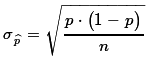and use that value to compute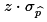.
5. Set the critical value (or values in the case of a two-sided test) such that it (they) mark the value(s) that is (are) that distance,, away from the proportion given by H0: p = a.
6. Then, we take a random sample of size n from the population.
7. We compute the sample proportion,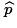.
8. If that proportionis more extreme than the critical value(s) then we say that "we reject H0 in favor of the alternate H1". If the sample proportion is not more extreme than the critical value(s) then we say "we have insufficient evidence to reject H0".
Attained significance Approach
1. We determine a sample size n. In doing this we need to be sure that both (n)(p)≥10 and (n)(1-p)≥10.
2. Also, we cannot sample more than 5% of the population. That means that the size of the population must be more than 20 times n.
3. Then, we take a random sample of size n from the population.
4. We compute the sample proportion,.
5. Compute.
6. Compute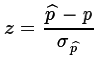.
7. Using the standard normal distribution, and taking into account the alternative hyposthesis, H1, so that we know if we are doing a one-tail or two-tail test, we compute the probability of getting the value z or a value more extreme than that.
8. If the resulting probability is smaller than or equal to the predetermined level of significance then we say that "we reject H0 in favor of the alternate H1". If the resulting probability is not less than the predetermined level of significance then we say "we have insufficient evidence to reject H0".
We will work our way through an example to see this. Assume that we have a population of values and that the members of that population fall into two groups, those with a certain characteristic and those without that characterisitic. In fact we will look at the population of M&M's in standard party packages of the candy. We will look at the proportion of the candies that are Green. Although it is true that there are 5 other colors of the candies (Red, Brown, Blue, Orange, and Yellow) we lump all those togethere as non-Green. Thus any particuar candy is either Green or non-Green.

I claim that the proportion of the population that is Green is 21%. That is, I claim that H0: p=0.21 is true. You do not believe me. You think the proportion of the population that is Green is not equal to 21%. That is, you believe that H1: p≠0.21 is true. Notice that you are not saying what the true proportion is, just that it is not 21%.

In order to test this difference of opinions, we agree to take a sample of size 75 from a large bin of M&M's that holds well over 4000 of the candies assembled from a random sample of party bags of M&M's. The size of the sample is important. We note that 75*0.21 = 15.75 ≥ 10 and 75*(1-0.21) = 59.25 ≥ 10. Furthermore, 75/4000 = 0.01875 so we are clearly sampling less than 5% of the population.

If the null hypothesis is true, p = 0.21, we know that the distribution of sample proportions from samples of size 75 will be sqrt(p*(1-p)/n) = sqrt(0.21*(1-0.21)/75) ≈0.0470319.

Critical value approach:

Our level of significance is 0.05. However, this is a two-tailed test (an extreme value either higher or lower than 0.21 would indicate that the null hypothesis is false). Therefore, we want to find, in the standard normal distribution, values for z that have 0.025 square units to the left of one value and 0.025 square units to the right of the other value. We could use the table, a calculator, or the computer to find those values. They turn out to be about -1.96 and 1.96, respectively. Figure 1 shows all the calculatorions that we have done so far.

Figure 1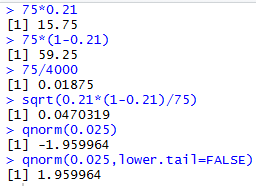Then we can find the critical values by finding the proportions that are z*σ away from the null hypothesis value, p. The calculations to do this are
```sigma <- sqrt(0.21*(1-0.21)/75)
z_left <- qnorm(0.025)
z_right <- qnorm(0.025,lower.tail=FALSE)
crit_low <-0.21 + z_left*sigma
crit_high <- 0.21 + z_right * sigma
crit_low
crit_high
```
The console view of those commands shown in Figure 2.

Figure 2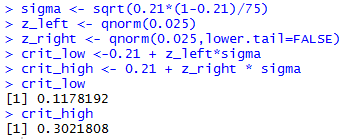The result is that our critical values are 0.1178 and 0.3022.

Now we take our actual sample, shown in Table 1 where the candy colors, Red, Green, Brown, Blue, orange, and Yellow, are represented by the values 1, 2, 3, 4, 5, and 6, respectively.
Now we need to find the proportion of the candies in the sample that are Green. To do this we count the number of 2's in the table. There are 10 such candies. The commands to generate the data, verify the data, and get the desired count, first using the built-in table() function and second using our make_freq_table() function are:
```gnrnd4(623127407,6997566)
L1
table(L1)
make_freq_table(L1)
```
The console view of those commands shown in Figure 3.

Figure 3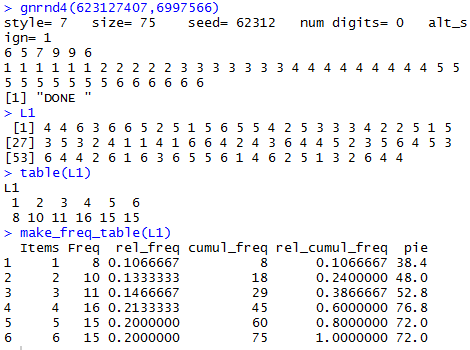Knowing that there are 10 Green candies out of 75 candies in the sample means that the proportion of Green candies is 10/75 or 0.1333, a value we would compute as 10/75 using the table() output or that we can read directly from the make_freq_table() output. Thus,=0.1333, a value that is neither below our low critical value, 0.1178 nor above our high critical value, 0.3022. Therefore we would conclude that we do not have sufficient evidence to reject H0 at the 0.05 level of significance.

Attained significance approach:

In this approach we still compute σ=sqrt(p*(1-p)/n), and we still take our sample, shown in Table 1, and we still compute. However, at that point we compute. Then using that value we find the area that is further away from 0.21 than that value of z. In this case, because this is a two-tail test, we need to add the area that is on the right, further away from 0.21 than our value of z was away on the left. But, we know that the distribution is normal and a normal distribution is symmetric. Therefore, the area on the right will be the same as the area on the left. To get the sum of the two areas we just need to double the area we found on the left. These computations are:
```sigma <- sqrt(0.21*(1-0.21)/75)
z <- (10/75-0.21)/sigma
# since the proportion is lower than 0.21
# look at the left tail
prob <- pnorm(z)
prob
prob*2
```
The console view of those commands shown in Figure 4.

Figure 4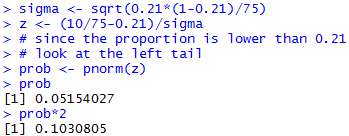From Figure 4 we see that the attained significance was 0.103, a value not less than the level of significance, 0.05, for the test. Therefore, we say we do not have sufficient evidence to reject the null hypothesis.

Changed problem:

Above we used a 5% level of significance. That meant that we were willing to make a Type I error 5% of the time. That is, even if H0: p = 0.21 is correct, if were repeat this process of taking samples of size 75 and base our decision to reject H0 or to not reject it on the proportion of Green candies in the sample, then we expect to incorrectly reject H0 about 5% of the time, that is, on average, 1 out of 20 times.

Now, look at the problem again, but this time we are willing to make a Type I error 12.5% of the time, on average, 1 out of 8 times. Using the same sample, the data in Table 1 we would have to redo some of the calculations for the critical value approach. In particular, we would need to do the calculations:
```sigma <- sqrt(0.21*(1-0.21)/75)
z_left <- qnorm(0.0625)
z_right <- qnorm(0.0625,lower.tail=FALSE)
crit_low <-0.21 + z_left*sigma
crit_high <- 0.21 + z_right * sigma
crit_low
crit_high
```
The console view of these commands appears in Figure 5.

Figure 5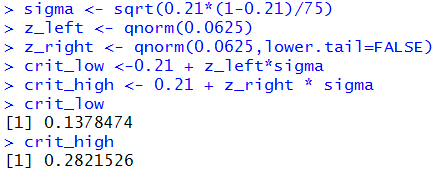The computation of `sigma` in Figure 5 is just a repeat of our earlier computation, first shown in Figure 2. The other computations are modified to get the two-tail values for the total of 0.125 square units outside the z-values, and then the computation of the actual critical values.

In this changed problem, because our sample produced a proportion of 0.1333 which is less than the new lower critical value, 0.1378, we would reject H0 at the 0.125 level in favor of H1.

You might note that using the attained siginificance approach we would still be using the information in Figure 4 and we would say that the attained significance 0.1031 is less than the new level, 0.125 so we would reject H0 at the 0.125 level in favor of H1.

A new problem:

We have a population of size 950 where some items in the population have with a characteristic and the rest do not. We have a null hypothesis that the proportion of the population that has that characterisitic is 0.25, that is 1 out of 4. We have an alternative hypothesis that the true proportion is greater than 0.25. We want to test H0: p = 0.25 against H1: p > 0.25 at the 0.0625 level of significance. This is a one-tail test. When we get around to taking a sample, in order to justify rejecting H0 in favor of H1 we will need to find the proportion of the sample with the characteristic to be significantly higher than 0.25. If we were to find the proportion in the sample to be 0.08 that might indicate that the 0.25 was wrong, but it would not suggest that the true value is actually higher than the 0.25 value.

We follow the same steps as we did above, except that this is a one-tail test. We decide on a sample size, n. We know that we need 0.25*n≥10 and (1-0.25)*n≥10. To meet that requirement, the smallest sample size that we can use is 40. To meet the requirement that we not sample more than 5% of the population we need to keep the sample size less than or equal to 0.05*950 = 47.5. We will settle on a sample size of n=45 to meet both restrictions.

```sigma <- sqrt(0.25*(1-0.25)/45)
sigma
z_right <- qnorm(0.0625,lower.tail=FALSE)
crit_high <- 0.25 + z_right * sigma
crit_high
```
The console view of these appears in Figure 6.

Figure 6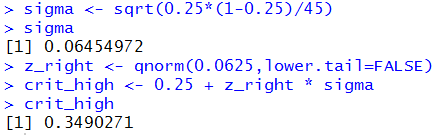From Figure 6 we see that the critical value is 0.349. Thus, when we take our sample of size 45 if the proportion of items with the specified characteristic is 0.349 or higher then we will reject the null hypothesis.

We take our random sample and find that it is the values given in Table 2.

 Just as a point of information, the specification of gnrnd4() used to produce Table 2 asks that function to produce a sample from a population that has 25% of the values having the characteristic. Now, gnrnd4() may not be perfect, but it does a reasonably good job of fullfilling that task. Therefore, if you reload this page over and over you should see that we do reject H0 about 6.25% of the time when it is really true.

Because we have different values foreach time we load the page, I cannot show you the exact R statements to compute the attained significance in this dynamic example. However, if the proportion was found to be 0.3111, the result of finding 14 of the 45 items having the characteristic, then we would use the commands:
```p_hat <- 0.3111
sigma <- sqrt(0.25*(1-0.25)/45)
sigma
z <- (p_hat-0.25)/sigma
z
prob <- pnorm(z, lower.tail=FALSE)
prob
```
which would produce the console output shown in Figure 7.

Figure 7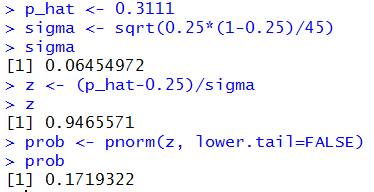I can tell you that for the specific sample shown in Table 2 where the proportion is

Yet another new problem:

We have a population of size 7589 where some items in the population have with a characteristic and the rest do not. We have a null hypothesis that the proportion of the population that has that characterisitic is 0.7, that is 7 out of 10. We have an alternative hypothesis that the true proportion is less than 0.7. We want to test H0: p = 0.7 against H1: p < 0.7 at the 0.03 level of significance. This is a one-tail test. When we get around to taking a sample, in order to justify rejecting H0 in favor of H1 we will need to find the proportion of the sample with the characteristic to be significantly lower than 0.7. If we were to find the proportion in the sample to be 0.85 that might indicate that the 0.7 was wrong, but it would not suggest that the true value is actually lower than the 0.7 value.

We follow the same steps as we did above, except that this is a one-tail test. We decide on a sample size, n. We know that we need 0.7*n≥10 and (1-0.7)*n≥10. To meet that requirement, the smallest sample size that we can use is 34. To meet the requirement that we not sample more than 5% of the population we need to keep the sample size less than or equal to 0.05*7589 = 379.45. This should not be a problem. We will settle on a sample size of n=35, a value that meets both restrictions.

```sigma <- sqrt(0.7*(1-0.7)/35)
sigma
z_left <- qnorm(0.03)
z_left
crit_low <- 0.7 + z_left * sigma
crit_low
```
The console view of some of our preliminary computations along with these commands appears in Figure 8.

Figure 8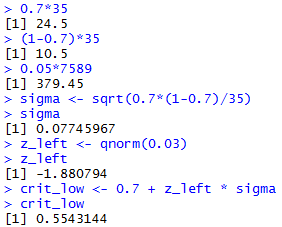From Figure 8 we see that the critical value is 0.5543. Thus, when we take our sample of size 35 if the proportion of items with the specified characteristic is 0.5543 or lower then we will reject the null hypothesis.

We take our random sample and find that it is the values given in Table 3.
Because we have different values foreach time we load the page, I cannot show you the exact R statements to compute the attained significance in this dynamic example. However, if the proportion was found to be 0.6, corresponding to having 21 of the items be a 2, then we would use the commands:
```p_hat <- 0.6
sigma <- sqrt(0.7*(1-0.7)/35)
sigma
z <- (p_hat-0.7)/sigma
z
prob <- pnorm(z)
prob
```
which would produce the console output shown in Figure 9.

Figure 9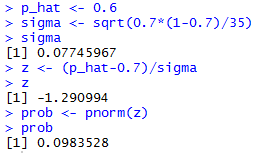I can tell you that for the specific sample shown in Table 3 where the proportion is

We have seen that we can use either the critical value or the attained significance approach to perform a test for the population proportion. There are not that many steps to either approach, but as has been the case in earlier hypothesis test pages, we might as well capture these steps in a function that we define in R. The quatities or directions tht we need to know to do the test are
1. the proportion stated in H0
2. the number of items in the sample that have the characteristic
3. the number of items in the sample
4. an indication of the statement of the alternative hypothesis, i.e., is it stated as ≠, <, or >
5. the specified level of significance.
If we have all of that then we can just go ahead and compute the result. Consider the following function:
```# Roger Palay copyright 2016-01-31
# Saline, MI 48176
#
hypoth_test_prop <- function(
H0_p, x, n, H1_type, sig_level=0.05)
{ # perform a hypothsis test for the proportion=H0_p
# based on finding x items out of the n items in
# the sample, where the alternative hypothesis  is
#      !=  if H1_type==0
#      <   if H1_type < 0
#      >   if H1_type > 0
# Do the test at sig_level significance,
# Do a little test to be sure we should do this.
if( H0_p*n < 10 )
{ return( "n*p must be >= 10") }
if( (1-H0_p)*n < 10 )
{ return( "n*(1-p) must be >=10" ) }
min_pop_size = 20*n
#get the s.d. of sample proportions
sigma = sqrt(H0_p*(1-H0_p)/n)
if(H1_type == 0 )
{ z_score <- abs( qnorm( sig_level/2 ) ) }
else
{ z_score <- abs( qnorm( sig_level ) ) }
decision <- "Reject"
samp_prop = x/n

z = ( samp_prop - H0_p)/sigma
if( H1_type == 0 )
{ crit_low  <- H0_p - z_score*sigma
crit_high <- H0_p + z_score*sigma
if( z < 0 )
{ attained <- 2*pnorm( z ) }
else
{ attained <- 2*pnorm(z, lower.tail=FALSE)}
alt <- paste("prop != ", H0_p)
}
else if (H1_type < 0 )
{ crit_low <- H0_p - z_score*sigma
crit_high <- "n.a."
attained <- pnorm( z )
alt <- paste("prop < ", H0_p)
}
else
{ crit_high <- H0_p + z_score*sigma
crit_low <- "n.a."
attained <- pnorm( z, lower.tail=FALSE )
alt <- paste("prop > ", H0_p)
}

if( attained > sig_level )
{ decision <- "Do Not Reject" }

result <- c(H0_p, alt, x, n, sig_level, sigma,
z_score, crit_low, crit_high,
samp_prop, z, attained, decision)
names(result) <- c("H0_p","H1","x","n","sig level",
"s.d. of prop",
"z-score","crit low", "crit_high",
"samp prop", "z", "attained",
"decision")
return( result )
}
```
As we have seen in earlier examples, this function is a bit longer than just repeating the statements that we used above. However, the function does a lot more than the mere statements that we have been using. For one thing, the function does an initial check on the appropriateness of the sample size. For another, the function takes care of all three possibilities for the alternative hypothesis. And, the function gives us all of the values that we might need.

We will apply the function to the three static cases shown above. First, for the case demonstrated in Figure 1  through 4, we would use the statement
```hypoth_test_prop(0.21,10,75,0,0.05)
```
The arguments for the command give the null hypothesis proportion (0.21), the number of items in the sample with the characteristic (10), the size of the entire sample (75), an indication that this is a two-tail test (0), and the significance level (0.05). The result of this command is shown in Figure 10.

Figure 10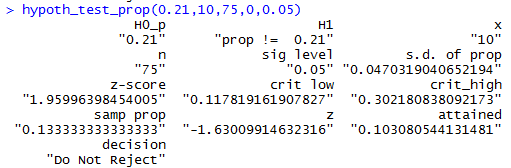The displayed values include the standard deviation of sample proportions and the z-score that we found in Figure 1, the low and high critical values that we found in Figure 2, the sample proportion that we found in Figure 3, and the attained significance that we found in Figure 4. Figure 10 includes the decision to not reject H0.

The second static example had a sample size of 45, 14 items had the characterisitic, the null hypothesis held that the true proportion is 0.25, the alternative hypothesis is that the proportion is greater than 0.25, and we want a 0.0625 level of significance. Using all of that we form the command
```hypoth_test_prop(0.25,14,45,1,0.0625)
```
the results of which are in Figure 11. (Note that the fourth argument, 1, could be any positive number to show that the alternative hypothesis is the "greater than" choice.)

Figure 11We see in Figure 11 values that we first computed in Figure 6 and Figure 7.

The third static example had a sample size of 35, 21 items had the characterisitic, the null hypothesis held that the true proportion is 0.7, the alternative hypothesis is that the proportion is less than 0.7, and we want a 0.03 level of significance. Using all of that we form the command
```hypoth_test_prop(0.7,21,35,-1,0.03)
```
the results of which are in Figure 12. (Note that the fourth argument, -1, could be any negative number to show that the alternative hypothesis is the "less than" choice.)

Figure 12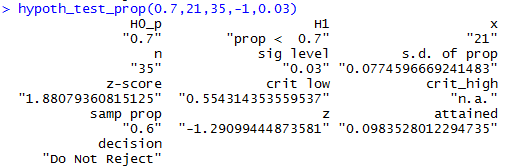We see in Figure 12 values that we first computed in Figure 8 and Figure 9.

Below is a listing of the R commands used in generating this page.
```source("../gnrnd4.R")
source("../make_freq_table.R")
75*0.21
75*(1-0.21)
75/4000
sqrt(0.21*(1-0.21)/75)
qnorm(0.025)
qnorm(0.025,lower.tail=FALSE)
sigma <- sqrt(0.21*(1-0.21)/75)
z_left <- qnorm(0.025)
z_right <- qnorm(0.025,lower.tail=FALSE)
crit_low <-0.21 + z_left*sigma
crit_high <- 0.21 + z_right * sigma
crit_low
crit_high

gnrnd4(623127407,6997566)
L1
table(L1)
make_freq_table(L1)

sigma <- sqrt(0.21*(1-0.21)/75)
z <- (10/75-0.21)/sigma
# since the proportion is lower than 0.21
# look at the left tail
prob <- pnorm(z)
prob
prob*2

sigma <- sqrt(0.21*(1-0.21)/75)
z_left <- qnorm(0.0625)
z_right <- qnorm(0.0625,lower.tail=FALSE)
crit_low <-0.21 + z_left*sigma
crit_high <- 0.21 + z_right * sigma
crit_low
crit_high

sigma <- sqrt(0.25*(1-0.25)/45)
sigma
z_right <- qnorm(0.0625,lower.tail=FALSE)
crit_high <- 0.25 + z_right * sigma
crit_high

p_hat <- 0.3111
sigma <- sqrt(0.25*(1-0.25)/45)
sigma
z <- (p_hat-0.25)/sigma
z
prob <- pnorm(z, lower.tail=FALSE)
prob

0.7*35
(1-0.7)*35
0.05*7589
sigma <- sqrt(0.7*(1-0.7)/35)
sigma
z_left <- qnorm(0.03)
z_left
crit_low <- 0.7 + z_left * sigma
crit_low

p_hat <- 0.6
sigma <- sqrt(0.7*(1-0.7)/35)
sigma
z <- (p_hat-0.7)/sigma
z
prob <- pnorm(z)
prob

source( "../hypo_prop.R")
hypoth_test_prop(0.21,10,75,0,0.05)
hypoth_test_prop(0.25,14,45,1,0.0625)
hypoth_test_prop(0.7,21,35,-1,0.03)
```